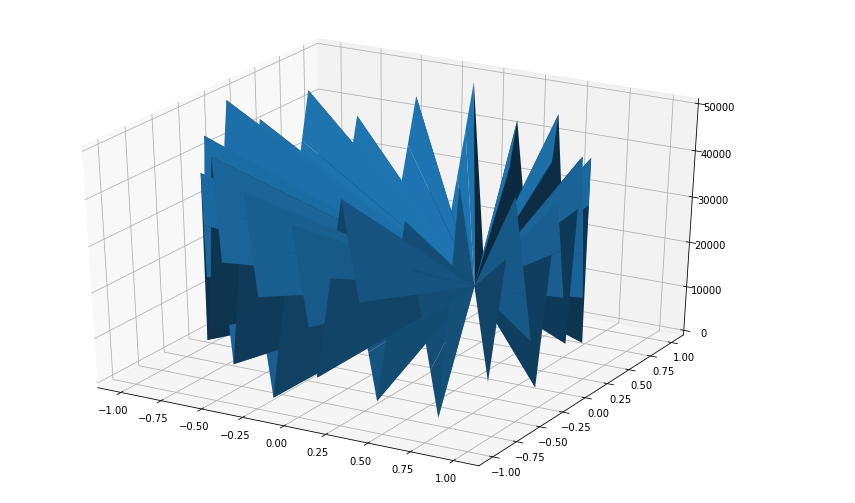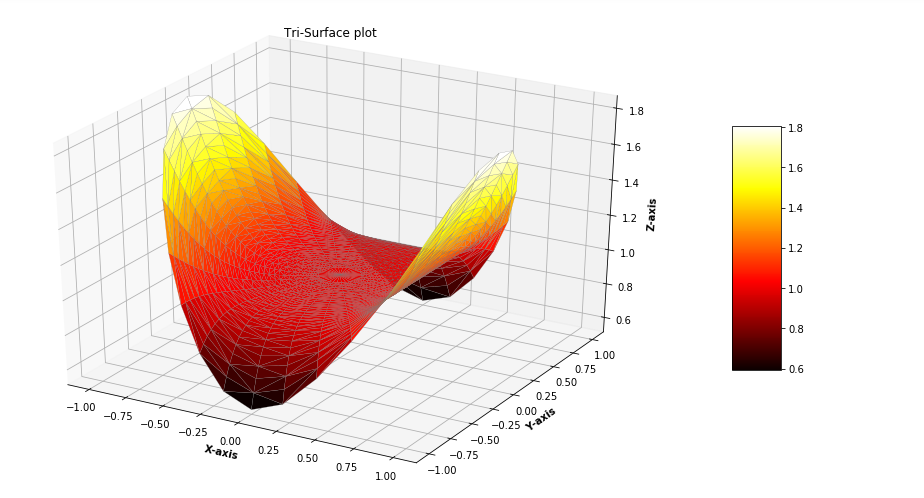Open in App
Not now

# Tri-Surface Plot in Python using Matplotlib

• Difficulty Level : Hard
• Last Updated : 11 Nov, 2021

A Tri-Surface Plot is a type of surface plot, created by triangulation of compact surfaces of finite number of triangles which cover the whole surface in a manner that each and every point on the surface is in triangle. The intersection of any two triangles results in void or a common edge or vertex. This type of plot is created where the evenly sampled grids are restrictive and inconvenient to plot. Generally Tri-Surface plots are created by calling ax.plot_trisurf() function of matplotlib library. Some of the attributes of the function are listed below:

Example 1: Let’s create a basic Tri-Surface plot using the ax.plot_trisurf() function.

## Python3

 `# Import libraries` `from` `mpl_toolkits ``import` `mplot3d` `import` `numpy as np` `import` `matplotlib.pyplot as plt`     `# Creating dataset` `z ``=` `np.linspace(``0``, ``50000``, ``100``)` `x ``=` `np.sin(z)` `y ``=` `np.cos(z) `   `# Creating figure` `fig ``=` `plt.figure(figsize ``=``(``14``, ``9``))` `ax ``=` `plt.axes(projection ``=``'3d'``)`   `# Creating plot` `ax.plot_trisurf(x, y, z,` `                ``linewidth ``=` `0.2``,` `                ``antialiased ``=` `True``);`   `# show plot` `plt.show()`

Output :Example 2 : For better understanding Let’s take another example.

## Python3

 `# Import libraries` `from` `mpl_toolkits.mplot3d ``import` `Axes3D  ` `import` `matplotlib.pyplot as plt  ` `import` `numpy as np  `     `# Creating radii and angles` `r ``=` `np.linspace(``0.125``, ``1.0``, ``100``)  ` `a ``=` `np.linspace(``0``, ``2` `*` `np.pi, ` `                ``100``,` `                ``endpoint ``=` `False``)  ` `  `  `# Repeating all angles for every radius  ` `a ``=` `np.repeat(a[..., np.newaxis], ``100``, axis ``=` `1``)  ` `  `  `# Creating dataset` `x ``=` `np.append(``0``, (r ``*` `np.cos(a)))  ` `y ``=` `np.append(``0``, (r ``*` `np.sin(a)))    ` `z ``=` `(np.sin(x ``*``*` `4``) ``+` `np.cos(y ``*``*` `4``)) ` `  `  `# Creating figure` `fig ``=` `plt.figure(figsize ``=``(``16``, ``9``))  ` `ax ``=` `plt.axes(projection ``=``'3d'``)  `   `# Creating color map` `my_cmap ``=` `plt.get_cmap(``'hot'``)` `  `  `# Creating plot` `trisurf ``=` `ax.plot_trisurf(x, y, z,` `                         ``cmap ``=` `my_cmap,` `                         ``linewidth ``=` `0.2``, ` `                         ``antialiased ``=` `True``,` `                         ``edgecolor ``=` `'grey'``)  ` `fig.colorbar(trisurf, ax ``=` `ax, shrink ``=` `0.5``, aspect ``=` `5``)` `ax.set_title(``'Tri-Surface plot'``)`   `# Adding labels` `ax.set_xlabel(``'X-axis'``, fontweight ``=``'bold'``) ` `ax.set_ylabel(``'Y-axis'``, fontweight ``=``'bold'``) ` `ax.set_zlabel(``'Z-axis'``, fontweight ``=``'bold'``)` `    `  `# show plot` `plt.show()`

Output:My Personal Notes arrow_drop_up
Related Articles